Thursday, May 30, 2013

David Mazza: The Transcendentality of Pi

By definition, the numberis the ratio of the circumference to the diameter of a circle. This ratio is the same for all circles.is an irrational number. It cannot be represented as the ratio of two integers, regardless of the choice of integers. Equivalently, it cannot be represented as an unending, periodic decimal.is also a transcendental number. It is not a root of any algebraic equation of the form

a0 + a1x + a2x2 + … + anxn = 0

where the ai are all rational numbers and n is finite. For comparison,is also an irrational number. Butis not
transcendental since it is a root of the equation

x2 - 2 = 0

Bothandare irrational, but onlyis transcendental. What makes the difference? One important argument is that a line of lengthcan beconstructed using classical techniques (i.e., using compass and straight-edge in a finite number of steps). But, because of the wayis defined, a line of lengthcannot be so constructed. (A curve can. Mark off a unit segment. Bisect the segment. Using the midpoint as center, scribe the appropriate circle. The circle has length.)

To illustrate, let us first consider. To make a construction that produces a line of this length, we begin with two unit-length segments placed end to end so that one segment is at right angles with the other. We then, connect the free ends to complete a right triangle. The new line has lengthNow, consider. A circle of unit diameter has its circumference =. Draw a unit circle, and locate its center. From the center produce a set of n radial lines each separated from its neighbor by an angle 2/n.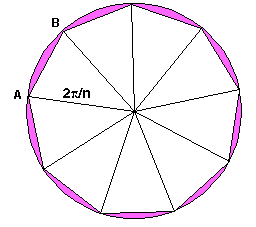Approximation for n = 9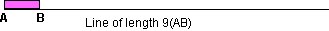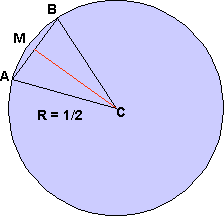Isoseles Triangle

Now, connect the ends with straight line segments to form a set of isosceles triangles. The sum of the lengths of these straight-line segments approaches the circumference of the circle as n approaches infinity (see figure for n = 9).
To construct a line of length, we have but to produce the length AB n times along any line. In the figure, n = 9, AB is a typical straight line segment completing an isosceles triangle, and Circumference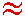9(AB) (see figure).
Now, let us use some algebra to calculate the length AB in the general case. We begin by redrawing part of our previous picture.
One isosceles triangle (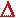ABC with one vertex at the center C and two more vertices on the circle at A and B) is selected (see figure). The angle subtended at C is 2/n. We wish to calculate the length AB, and then to estimate how good an approximation the value n(AB) is to the actual circumference of the circle.

The line CM bisects the angle 2/n, and meets the line AB at right angles. Thus, the triangle CMA is a right triangle, and the length AM = ½(AB).

AM/(Radius) = AM/(½) = sin (/n)AM = ½ sin (/n)

& AB = sin (/n).

Finally, n(AB) = n sin (/n).

Since the actual circumference of the circle is, we now write

n(AB) = {n sin (/n)}/{(sin (/n))/(/n)}{(sin ())/()}

where/n.

The value n(AB) differs fromby the multiplicative factor (sin ())/(), with=/n.

Notice that, in the limit as n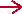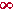0 and (sin ())/(1. The value n(AB) does indeed approachin the limit

But, while the limitexists, the actual function f() = (sin ())/() does not exist at= 0. It becomes the indeterminate form 0/0.

Thus, although n(AB) may approach the actual circumference to any arbitrary precision we might desire, the actual value n(AB) =can never be obtained from any construction of the type outlined above.

---------

http://www.grc.nasa.gov/WWW/k-12/Numbers/Math/Mathematical_Thinking/transcendentality_of_p.htm